Differential Equation MCQ Level - 2

# Differential Equation MCQ Level - 2

Test Description

## 10 Questions MCQ Test Topic wise Tests for IIT JAM Physics | Differential Equation MCQ Level - 2

Differential Equation MCQ Level - 2 for IIT JAM 2023 is part of Topic wise Tests for IIT JAM Physics preparation. The Differential Equation MCQ Level - 2 questions and answers have been prepared according to the IIT JAM exam syllabus.The Differential Equation MCQ Level - 2 MCQs are made for IIT JAM 2023 Exam. Find important definitions, questions, notes, meanings, examples, exercises, MCQs and online tests for Differential Equation MCQ Level - 2 below.
Solutions of Differential Equation MCQ Level - 2 questions in English are available as part of our Topic wise Tests for IIT JAM Physics for IIT JAM & Differential Equation MCQ Level - 2 solutions in Hindi for Topic wise Tests for IIT JAM Physics course. Download more important topics, notes, lectures and mock test series for IIT JAM Exam by signing up for free. Attempt Differential Equation MCQ Level - 2 | 10 questions in 45 minutes | Mock test for IIT JAM preparation | Free important questions MCQ to study Topic wise Tests for IIT JAM Physics for IIT JAM Exam | Download free PDF with solutions
 1 Crore+ students have signed up on EduRev. Have you?
Differential Equation MCQ Level - 2 - Question 1

### (D2 - 1)y = x sin x + (1 + x2)ex, will be satisfied by which of the following functions?

Detailed Solution for Differential Equation MCQ Level - 2 - Question 1

The given differential equation is,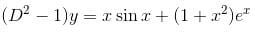The auxiliary equation is
m2 – 1 = 0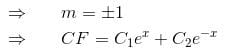or C1ex + C2ex      (as C1C2 are arbitrary constant)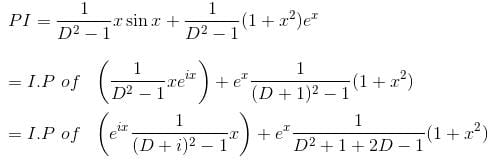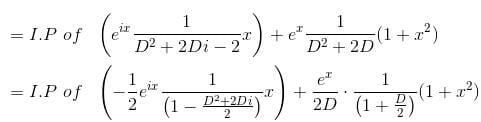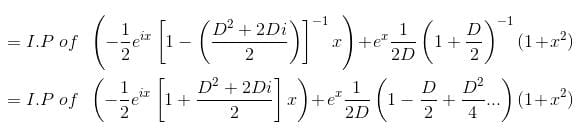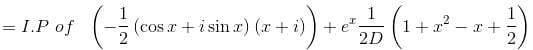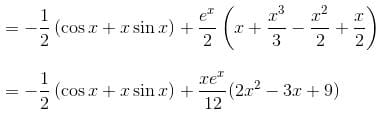Hence, y = CF + PI gives the required functions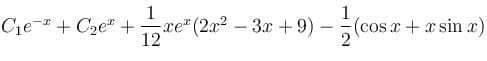Differential Equation MCQ Level - 2 - Question 2

### If [(x + 1)2 f(x) – g(x)] is the particular integral of the differential equation,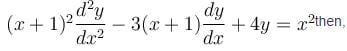Detailed Solution for Differential Equation MCQ Level - 2 - Question 2

Let x + 1 = ez so that z = log (x + 1) and x = ez – 1.

The given equation becomes

[D(D – 1) – 3D + 4]y = (ez –1)2D = d/dz
or    (D2 – 4D + 4)y = e2z – 2ez + 1
The auxiliary equation is m2 – 4m + 4 = 0
⇒   (m – 2)2 = 0
∴   C.F. = [(C1 + C2z)e2z = [C1 + C2log (x + 1)] (x +1)2.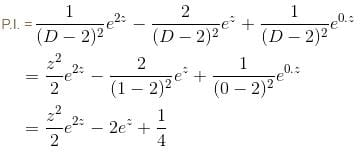∴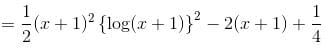The correct answer is: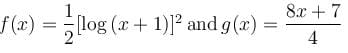Differential Equation MCQ Level - 2 - Question 3

### The general solution of the differential equation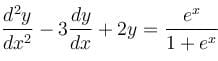will be :

Detailed Solution for Differential Equation MCQ Level - 2 - Question 3

The auxiliary equation isLet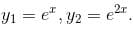Their wronskian is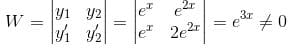P.I. = u1y1 + u2y2, where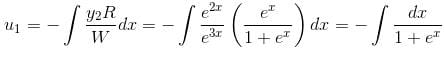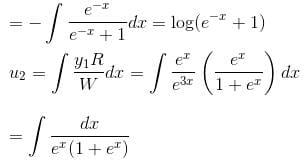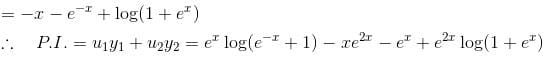Hence y = C.F. + P.I. is the complete solution.

The correct answer is: y = ex log (ex + 1) – xe2x – ex + e2x log(ex + 1) + C1ex + C2e2x

Differential Equation MCQ Level - 2 - Question 4

Given that the differential equation (axy2 + by)dx + (bx2y + ax)dy = 0, (a ≠ b) is not exact. If xmyn is an integrating factor of the given equation, then

Detailed Solution for Differential Equation MCQ Level - 2 - Question 4

Multiplying the given differential equation with xmyn and comparing the equation with

M dx + N dy = 0
We have,
M = xmyn (axy2 + by)
= axm+1yn+2 + bxmyn+1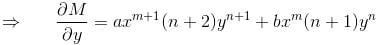∵  The equation is exact now,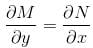Comparing coefficients we get,

a(n + 2) = b(m + 2)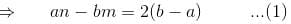and    b(n +1) = a(m +1)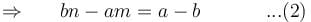abn – b2m = 2(b – a)b          ...(3)
abn – a2m = (a – b)a            ...(4)
Solving (3) and (4), we get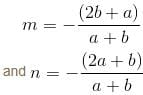The correct answer is: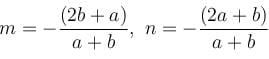Differential Equation MCQ Level - 2 - Question 5

The slope of the tangent at the point (xy) to a curve passing through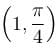is given by,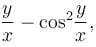then the equation of the curve is :

Detailed Solution for Differential Equation MCQ Level - 2 - Question 5

We know that the slope is given by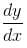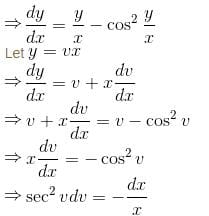Integrating both sides,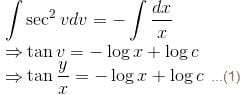Given that the curve passes through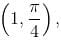so it will satisfy the equation of the curve.
Putting x = 1 and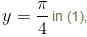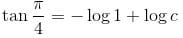1 = log c
⇒ c = e
Putting c = e in (1),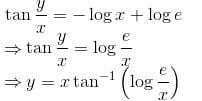is the required curve.

The correct answer is: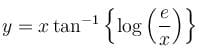Differential Equation MCQ Level - 2 - Question 6

The general solution of the equation, (D2 - 6D + 8)y = (e2x - 1)2 + sin3x will be :

Detailed Solution for Differential Equation MCQ Level - 2 - Question 6

The auxiliary equation is m2 – 6m + 8 = 0 or (m – 4)(m – 2) =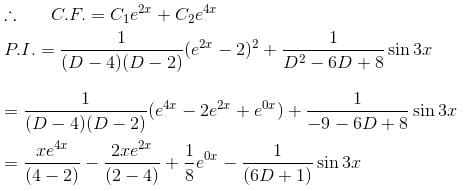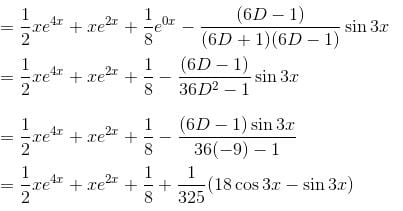Hence the required solution is y = C.F. + P.I.

The correct answer is: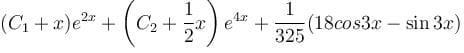Differential Equation MCQ Level - 2 - Question 7

If (x, y) is the solution to the system of equations,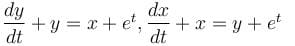then x is equal to :

Detailed Solution for Differential Equation MCQ Level - 2 - Question 7

The given equation can be written as
(D – 1)x + (2D + 1)y = 0          ...(1)
2(D + 1)x + (D + 1)y = 3et     ...(2)

Multiplying (1) by 2(D + 1), and (2) by (D – 1) and subtracting,
{2(D + 1)(2D + 1) – (D2 – 1)}y = –3(D – 1)et
= –3 (–et – et) = 6et

or (D2 + 2D + 1)y = 2et          ...(3)
C.F. = (a + bt)et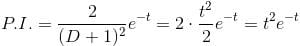The solution of (3) is y = (a + bt + t2)et
The correct answer is: (a + bt)et + tet

Differential Equation MCQ Level - 2 - Question 8

The curve orthogonal to the curve x2 + 2xy – y2 = k would be :

Detailed Solution for Differential Equation MCQ Level - 2 - Question 8

The given curve is,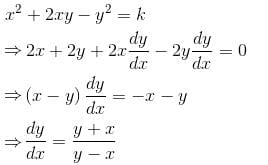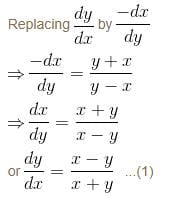which is a homogeneous equation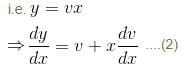from (1) and (2)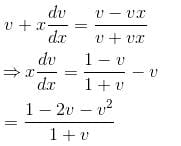Integrating  both sides,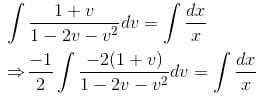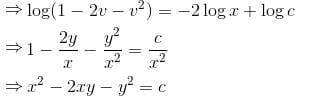The correct answer is: x2 – 2xy – y2 = k

Differential Equation MCQ Level - 2 - Question 9

The particular integral of the differential equation,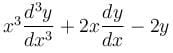= x2 log x + 3x is

Detailed Solution for Differential Equation MCQ Level - 2 - Question 9

This is a homogeneous linear equation.

Putting z = log x ⇔ x = ez, the given equation becomes

[D (D – 1) (D – 2) + 2D – 2] = ze2z + 3ez

or    (D3 – 3D2 + 4D – 2) = ze2z + 3z2

The auxiliary equation is m3 – 3m2 + 4m – 2 = 0

⇒  (m – 1) (m2 – 2m + 2) = 0
⇒ m =1, 1 ± i
∴   C.F.
C1ez + ez (C2 cos z + C3 sin z)
x[C1 + C2 cos (log x) + C3 sin (log x)]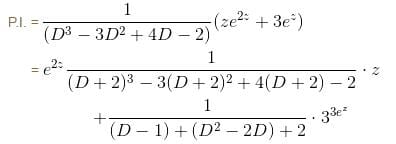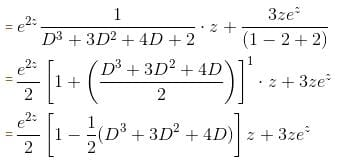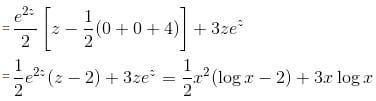The correct answer is: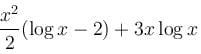Differential Equation MCQ Level - 2 - Question 10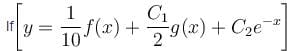is the solution of the differential equation, D2y + 1 (1 - cot x) Dy - y cot x = sin2 x then

Detailed Solution for Differential Equation MCQ Level - 2 - Question 10

We have P = 1 – cot xQ = –cot x and so 1 – P + Q = 0.

Thus ex is an integral of D2y + (1 – cot xDy – y cot x = 0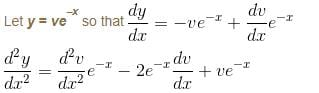The given equation now becomes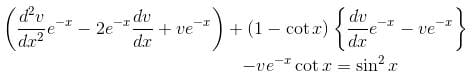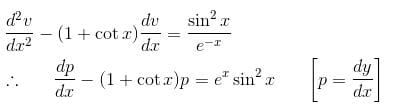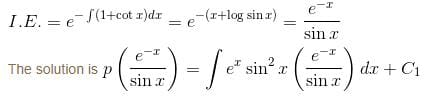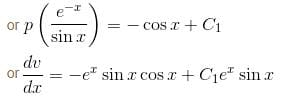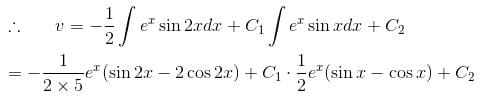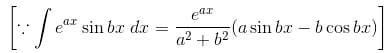Hence,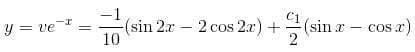is the required solution.

The correct answer is: f(x) = 2 cos 2x – sin 2x and g(x) = sin x – cos x

## Topic wise Tests for IIT JAM Physics

217 tests
Information about Differential Equation MCQ Level - 2 Page
In this test you can find the Exam questions for Differential Equation MCQ Level - 2 solved & explained in the simplest way possible. Besides giving Questions and answers for Differential Equation MCQ Level - 2, EduRev gives you an ample number of Online tests for practice

## Topic wise Tests for IIT JAM Physics

217 tests(Scan QR code)